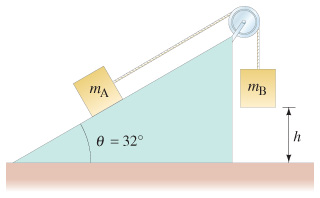# Problem: Two masses are connected by a string as shown in the figure. mA exttip{m_{ m A}}{m_A}= 3.6 kg rests on a frictionless inclined plane, while mB exttip{m_{ m B}}{m_B}= 4.6 kg is initially held at a height of h exttip{h}{h}= 0.75 m above the floor.(a) If mB is allowed to fall, what will be the resulting acceleration of the masses?(b) If the masses were initially at rest, use the kinematic equations to find their velocity just before mB hits the floor.(c) Use conservation of energy to find the velocity of the masses just before mB hits the floor.

🤓 Based on our data, we think this question is relevant for Professor White's class at UTA.

###### Problem Details

Two masses are connected by a string as shown in the figure. mA = 3.6 kg rests on a frictionless inclined plane, while mB = 4.6 kg is initially held at a height of h = 0.75 m above the floor.
(a) If mB is allowed to fall, what will be the resulting acceleration of the masses?
(b) If the masses were initially at rest, use the kinematic equations to find their velocity just before mB hits the floor.
(c) Use conservation of energy to find the velocity of the masses just before mB hits the floor.